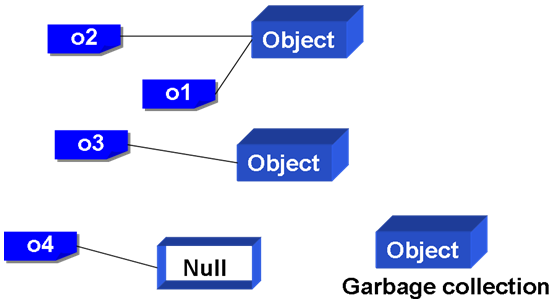# Java SE 6 技術手冊

## 7.2 關於方法

``````static String valueOf(boolean b)
static String valueOf(char c)
static String valueOf(char[] data)
static String valueOf(char[] data, int offset, int count)
static String valueOf(double d)
static String valueOf(float f)
static String valueOf(int i)
static String valueOf(long l)
static String valueOf(Object obj)
``````

``````public class SomeClass {
// 以下重載了someMethod()方法
public void someMethod() {
// ...
}
public void someMethod(int i) {
// ...
}
public void someMethod(float f) {
// ...
}
public void someMethod(int i, float f) {
// ...
}
}
``````

``````public class SomeClass {
public int someMethod(int i) {
// ...
return 0;
}
public double someMethod(int i) {
// ...
return 0.0;
}
}
``````

``````public class OverloadTest {
public static void main(String[] args) {
someMethod(1);
}

public static void someMethod(int i) {
System.out.println("int 版本被呼叫");
}

public static void someMethod(Integer integer) {
System.out.println("Integer 版本被呼叫");
}
}
``````

``````someMethod(new Integer(1));
``````

• 找尋在還沒有裝箱動作前可以符合引數個數與型態的方法
• 嘗試裝箱動作後可以符合引數個數與型態的方法
• 嘗試設有「不定長度引數」並可以符合的方法
• 編譯器找不到合適的方法，回報編譯錯誤

### 7.2.2 不定長度引數

``````System.out.printf("%d", 10);
System.out.printf("%d %d", 10, 20);
System.out.printf("%d %d %d", 10, 20, 30);
``````

#### 範例 7.10 MathTool.java

``````public class MathTool {
public static int sum(int... nums) { // 使用...宣告參數
int sum = 0;
for(int num : nums) {
sum += num;
}
return sum;
}
}
``````

#### 範例 7.11 TestVarargs.java

``````public class TestVarargs {
public static void main(String[] args) {
int sum = 0;

sum = MathTool.sum(1, 2);
System.out.println("1 + 2 = " + sum);

sum = MathTool.sum(1, 2, 3);
System.out.println("1 + 2 + 3 = " + sum);

sum = MathTool.sum(1, 2, 3, 4, 5);
System.out.println("1 + 2 + 3+ 4+ 5 = " + sum);
}
}
``````

``````1 + 2 = 3
1 + 2 + 3 = 6
1 + 2 + 3+ 4+ 5 = 15
``````

``````public void someMethod(int arg1, int arg2, int... varargs) {
// ....
}
``````

``````public void someMethod(int... varargs, int arg1, int arg2) {
// ....
}
``````

``````public void someMethod(int... varargs1, int... varargs2) {
// ....
}
``````

``````public void someMethod(SomeClass... somes) {
// ....
}
``````

### 7.2.3 遞迴方法

「遞迴」（Recursion）是在方法中呼叫自身同名方法，而呼叫者本身會先被置入記憶體「堆疊」（Stack）中，等到被呼叫者執行完畢之後，再從堆疊中取出之前被置入的方法繼續執行。堆疊是一種「先進後出」（First in, last out）的資料結構，就好比您將書本置入箱中，最先放入的書會最後才取出。 Java 支援遞迴，遞迴的實際應用很多，舉個例子來說，求最大公因數就可以使用遞迴來求解，範例 7.12 是使用遞迴來求解最大公因數的一個實例。

#### 範例 7.12 UseRecursion.java

``````import java.util.Scanner;

public class UseRecursion {
public static void main(String[] args) {
Scanner scanner = new Scanner(System.in);

System.out.println("輸入兩數:");
System.out.print("m = ");
int m = scanner.nextInt();

System.out.print("n = ");
int n = scanner.nextInt();

System.out.println("GCD: " + gcd(m, n));
}

private static int gcd(int m, int n) {
if(n == 0)
return m;
else
return gcd(n, m % n);
}
}
``````

``````輸入兩數:
m = 10
n = 20
GCD: 10
``````

``````private static int gcd(int m, int n) {
int r;
while(n != 0) {
r = m % n;
m = n;
n = r;
}
return m;
}
``````

### 7.2.4 垃圾收集``````public GcTest(String name) {
this.name = name;
System.out.println(name + "建立");
}

// 物件回收前執行
protected void finalize() {
System.out.println(name + "被回收");
}
``````

}.

#### 範例 7.14 UseGC.java

``````public class UseGC {
public static void main(String[] args) {
System.out.println("請按Ctrl + C終止程式........");

GcTest obj1 = new GcTest("object1");
GcTest obj2 = new GcTest("object2");
GcTest obj3 = new GcTest("object3");

// 令名稱不參考至物件
obj1 = null;
obj2 = null;
obj3 = null;

// 建議回收物件
System.gc();

while(true); // 不斷執行程式
}
}
``````

``````請按Ctrl + C終止程式........
bject1建立
bject2建立
bject3建立
bject3被回收
bject2被回收
bject1被回收
``````